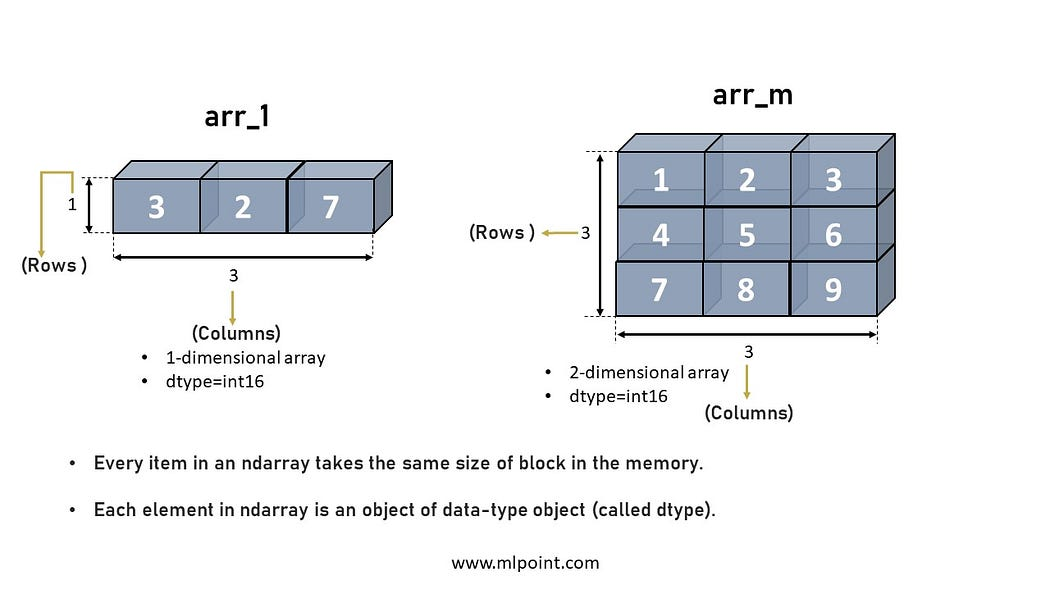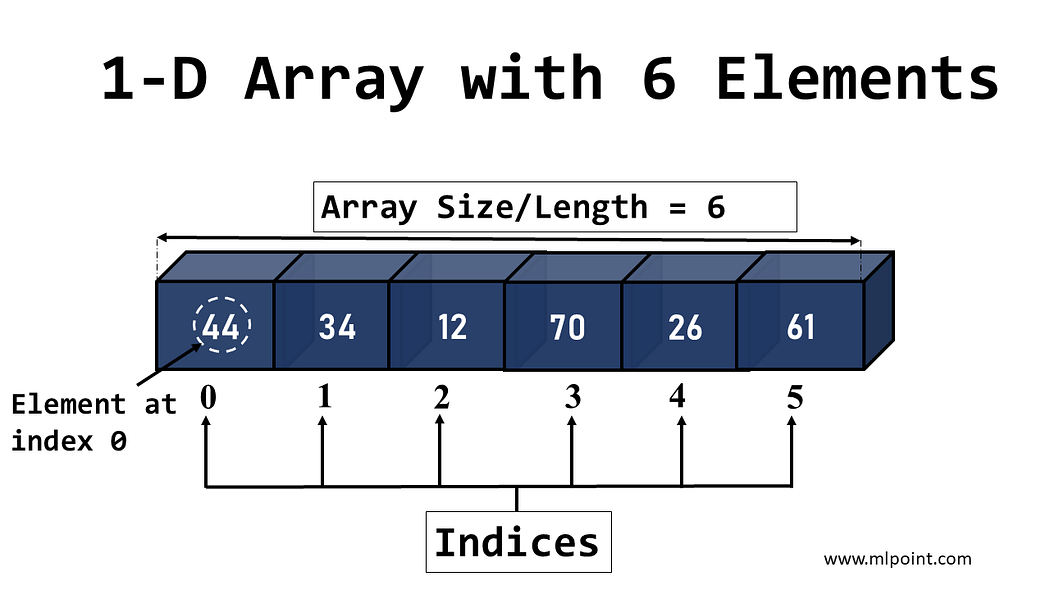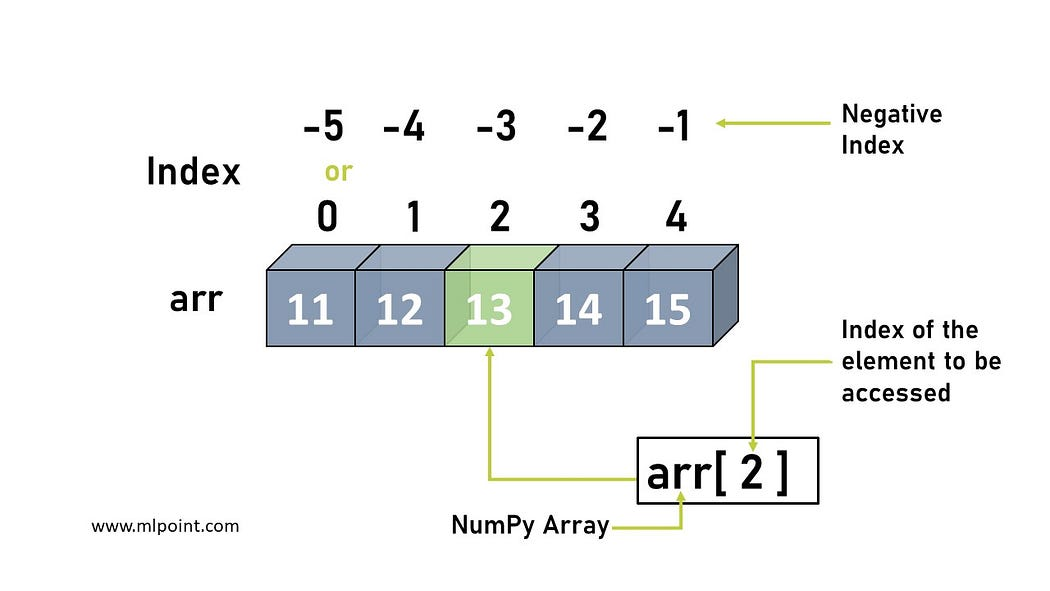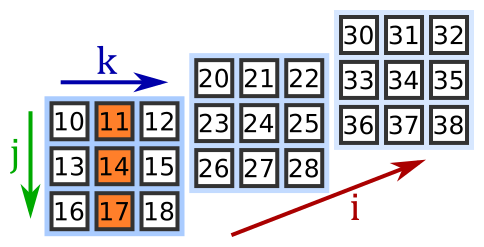# Numpy Interview Questions & Answers

NumPy(Numerical Python) is an open-source library of Python. It is designed to perform complex mathematical, image processing, quantum computing, and statistical operations, etc., on matrices and multidimensional arrays. The NumPy N-dimensional arrays are used to perform indexing, slicing, splitting, joining, reshaping, and other complex manipulations.

These robust in-built functions make NumPy a great choice for data science projects. As the demand for data scientists and jobs in machine learning is rising, it is a wise choice to learn NumPy and carve a golden career path along with it.

## Basic NumPy Interview Questions and Answers

### 1. Name a few use cases where NumPy is useful.

• To perform complex mathematical computations on arrays
• To use multi-dimensional arrays and matrices in operations
• To execute trigonometric, statistical, and algebraic functions
• To execute transforms and methods for shape manipulation
• To generate random numbers
• To add/ delete/ sort/ split arrays

### 2. How many dimensions can a NumPy array have?

In NumPy, an array can have N-dimensions, and is called a ndarray.

• Ndarray is a multidimensional container which contains elements of the same type and size.
• The number of dimensions in a ndarray is also known as ‘rank’.
• A tuple of integers called ‘shape’ defines the size of the array in each dimension.
• The data type of elements in ndarray is defined by a  ‘dtype’ object.Example:

```2D ndarray with size 2X3.

example = np.array([[1, 2, 3], [4, 5, 6]], np.int32)
type(example)
<class 'numpy.ndarray'>
example.shape(2, 3)
example.dtype
dtype('int32')```

### 3. What is negative indexing in NumPy arrays?

Usually, the indices start from zero(0), continuing to 1,2,3, and so onHowever in NumPy the elements can be accessed starting from the end of the array too. Negative indexing refers to the act of indexing from the end of the list starting at -1.```Example:
Print the last element from the 2nd dimension:

import numpy as np

example = np.array([[1,2,3,4,5], [6,7,8,9,10]])
print('Last element from 2nd dim: ', example[1, -1])

Output
10```

### 4. How are NumPy Arrays better than Lists in Python?### 5. Explain the data types supported by  NumPy.

• numpy.bool_ : bool
• numpy.byte : signed char
• numpy.ubyte : unsigned char
• numpy.short : short
• numpy.ushort : unsigned short
• numpy.intc : int
• numpy.uintc : unsigned int
• numpy.int_ : long
• numpy.uint : unsigned long
• numpy.longlong :  long long
• numpy.ulonglong : unsigned long long
• numpy.half / numpy.float16 : Half precision float
• numpy.single : float
• numpy.double : double
• numpy.longdouble : long double
• numpy.csingle : float complex
• numpy.cdouble : double complex
• numpy.clongdouble : long double complex

More details and examples are here.

## Intermediate NumPy interview questions and answers

### 1. Explain the operations that can be performed in NumPy.

Operations on 1-D arrays in NumPy:

```Example:

array1= np.array([1,2,3,4,5])
array2= np.array([1,2,3,4,5])
array3= array1 + array2
array3

Output - array([1,4,6,8,10])  ```
• Multiply : Multiplies the elements with a number, depicted by ‘*’.
• Power: Square the elements in an array by using ‘**’.
```Example:

array1**2
array1

Output - array1([1,4,9,16,10])```

To use a higher power such as cube, use below syntax.

```np.power(array1,3)

array1

Output - array1([1,8,27,64,1000])```

• Conditional Expressions: Compare the array elements using a conditional expression.
```Example:

array5 = array1 >= 3

array5

Output - array([False,False, True, True , True])```

Operations on 2-D arrays in NumPy:

```Example:

A = np.array([[3,2],[0,1]])

B = np.array([[3,1],[2,1]])

A+B gives output as-

array([[6, 3],

[2, 2]])```

• Normal multiplication – Multiples the arrays element-wise.
```Example:

A*B gives output as-

array([[5, 4],

[2, 3]])  ```
• Matrix Multiplication- Uses ‘@’ to perform matrix product/multiplication.
```Example:

A@B gives output as-

array([[13,  5],

[ 2,  1]])```

### 2. Why is the shape property used in NumPy?

The shape property is used to get the number of elements in each dimension of a  NumPy array.

```Example:

example = np.array([[1, 2, 3, 4], [5, 6, 7, 8]])

print(example.shape)

Output:

(2,4)

```

This means there are 2 dimensions and each dimension has 4 elements.

### 3. What is array slicing in NumPy?

Through slicing a portion of the array is selected, by mentioning the lower and upper limits. Slicing creates views from the actual array and does not copy them.

Syntax:

The basic slice syntax is i:j:k where i is the starting index, j is the stopping index, and k is the step (k≠0).

```Example 1-D array:

x = np.array([0, 1, 2, 3, 4, 5, 6, 7, 8, 9])

x[1:7:2]

Output

array([1, 3, 5])

Example 2-D array:

a2 = np.array([[10, 11, 12, 13, 14],

[15, 16, 17, 18, 19],

[20, 21, 22, 23, 24],

[25, 26, 27, 28, 29]])

print(a2[1:,2:4])```

This starts from row 1:(till the end of the array) and columns 2:4(2 and 3).

```Example 3-D array:

a3 = np.array([[[10, 11, 12], [13, 14, 15], [16, 17, 18]],

[[20, 21, 22], [23, 24, 25], [26, 27, 28]],

[[30, 31, 32], [33, 34, 35], [36, 37, 38]]])

print(a3[:2,1:,:2])```

This statement makes a selection as shown below-

• Planes : first 2 planes
• Rows:  last 2 rows
• Columns: first 2 columns### 4. How is the seed function used in NumPy?

• The seed function sets the seed(provides input) of a pseudo-random number generator in NumPy.
• By pseudo-random we mean that the numbers appear as randomly generated but actually are predetermined through algorithms.
• The numpy.random.seed or np.random.seed function can not be used alone and is used together with other functions.
```Example:

# seed random number generator

seed(1)

# generate some random numbers

print(rand(3))```

### 5. How to convert the data type of an array in NumPy?

The data type(dtype) of an array can be changed using the function numpy.astype().

```Example: Change the array of floats to an array of integers.

arr = np.array([1.3, 2.2, 3.1])

newarr = arr.astype('i')

print(newarr)

print(newarr.dtype)

Output:

[1 2 3]

int32```

### 6. What is the difference between copy and view in NumPy?

Copy

• Returns a copy of the original array.
• Do not share the data or memory location with the original array.
• Any modifications made in the copy will not get reflected in the original.
```Example:

import numpy as np

arr = np.array([20,30,50,70])

a= arr.copy()

#changing a value in copy array

a = 5

print(arr)

print(a)

Output:

[20 30 50 70]

[ 5 30 50 70]```

View

• Returns a view of the original array.
• Does use the data and memory location of the original array.
• Any modifications made in the copy will get reflected in the original.
```Example:

import numpy as np

arr = np.array([20,30,50,70])

a= arr.view()

#changing a value in original array

arr = 100

print(arr)

print(a)

Output:

[100 30 50 70]

[100 30 50 70]```